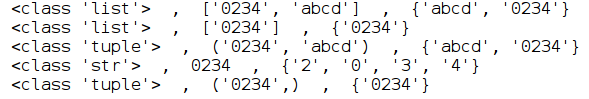## 在Python里的字符串、列表、元组、集合之间转换的一个不为人知的细节

``````a = ['0234','abcd']
seta = set(a)

b = ['0234']
setb = set(b)

c = ('0234','abcd')
setc = set(c)

d = ('0234')
setd = set(d)

e= tuple(b)
sete = set(e)

print(type(a), ' , ', a,' , ',seta)
print(type(b), ' , ', b,' , ',setb)
print(type(c), ' , ', c,' , ',setc)
print(type(d), ' , ', d,' , ',setd)
print(type(e), ' , ', e,' , ',sete)``````1.通过()和tuple这两种方式定义元组，得到的结果可能是不同的，比如上面例子中的用一个字符串以()和tuple定义元组，结果却一个仍是字符串，一个是元组

2.一个字符串转换成集合，会把字符串拆分成字符并作为集合的元素，而用字符串组成的列表或元组，转换成集合时，却不会拆分字符串，而是把字符串作为集合的一个元素